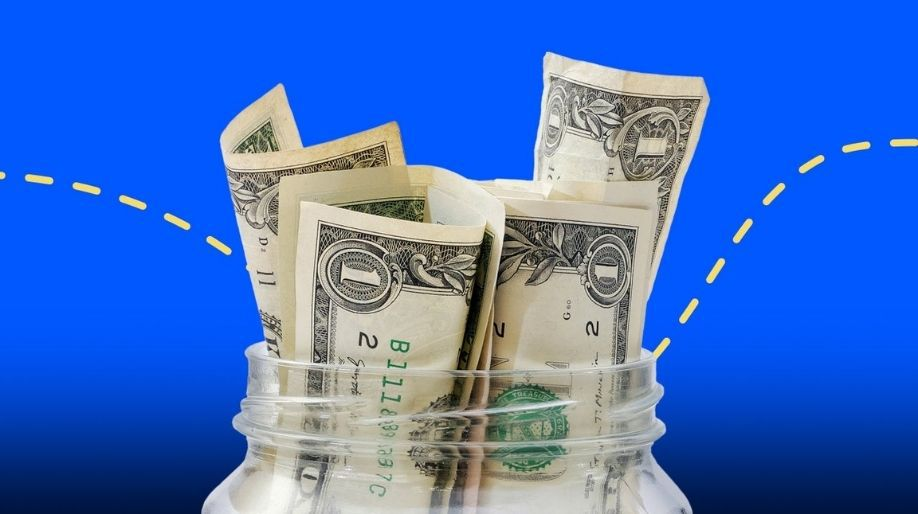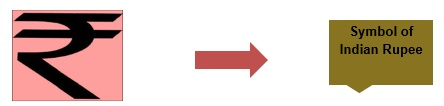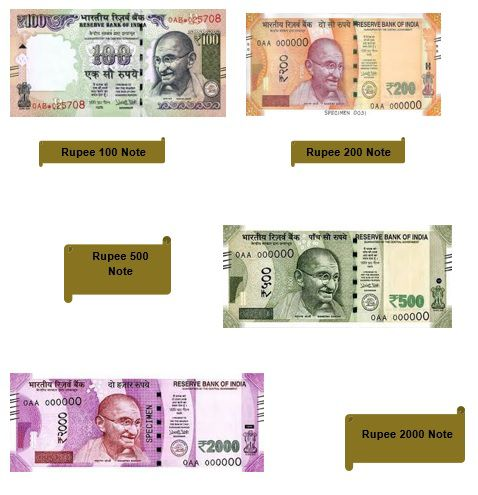Register for Maths, Science, English, Reasoning Olympiad Exams Register here | Book Free Demo for Live Olympiad Classes here | Check Olympiad Exam Dates here | Buy Practice Papers for IMO, IOM, HEO, IOEL etc here | Login here to participate in all India free mock test on every Saturday

# MoneyWhat is money? When we all go for shopping we buy some stuff but how do we pay for it? We all know the answer “We pay using money". We all it daily for our needs but what money is and what are its uses and applications? Let’s just find out.

• The currency used in any country is called as money. Like in India we use Indian Rupee (INR) which is the currency of India and hence money in simple language. Thus the coins, the notes of different denominations are all money.

• RUPEE has been derived from a Sanskrit word ‘RAUPYA’ which means silver coins.

The currencies used in different countries are mentioned in the table

 Country Currency United states of America (USA) US Dollars(USD) Canada Canadian Dollar Nepal Nepalese Rupee Pakistan Pakistani Rupee China Renminbi

Different Denominations

The different types of notes and coins used across the whole country centralized by the Government are called as denominations of the money or currency.

• The different denominations of The Indian Rupee are ₨ 5, ₨ 10, ₨ 20, ₨ 50, ₨ 100, ₨ 200, ₨ 500, ₨ 2000.
• n our Indian currency system 1 Rupee = 100 Paisa.
•  It means 1 Paisa = 0.01 Rupee.
•  At earlier times we also had 25 paisa and 50 paisa coins.
•  We get our currency in form of notes as mentioned above and also in the form of coins like Rs 1 coin, Rs 2 coin, Rs 5 coin, Rs 10 coin.
•  1 Rupee = 25 Paisa x 4 , hence 4 coins of 25 Paisa will make a rupee.Let’s Identify the Pictures

[Image_Link]../../assets/images/maths_square/M03MON02.jpgRole of Money in Mathematics

Like everywhere else Maths has very great use and importance in the field of money and money related problems. We use it while shopping, while calculating bills, while receiving money from someone or giving money to someone.

• Thus Maths and Money are inter-related to each other and we need both of them in our daily life.

Let’s just understand it in a better way with the help of an example

Example 1: A shopkeeper is selling Ghee at Rs 230/kg. If Aman has to buy 5 kg of Ghee, How much will he have to pay to the shopkeeper?

Solution:

Rate of Ghee = ₨ 230/kg

Quantity of Ghee which Aman has to buy = 5 kg

Therefore for 5kg cost of Ghee = ₨ 230 x 5 = ₨ 1150.

Hence Aman has to pay ₨ 1150 to the shopkeeper.

Example 2: If in the above example if Aman gave ₨ 2000 note to the shopkeeper. How much cash would he receive back?

Solution:

Amount given by Aman to the shopkeeper = ₨ 2000

Cost of 5 kg Ghee = Rs 1150

= (Rs2000 – Rs1150) = Rs 850.

• These types are problems related to money are dealt with using mathematics.

Let’s just go through some more examples

Example 3: Ravi is going for a dinner with 4 of his friends. After having dinner the bill came out to be Rs 2356. But Ravi has only Rs 1980. So how much money he has to borrow from his friends?

Solution:

Total amount of dinner = Rs 2356

Amount Ravi has = Rs1980

Therefore amount of money Ravi has to ask his friends is

= Rs 2356 – Rs 1980 = Rs 376.

Example 4: Convert Rs 23.57 into Paisa.

Solution- As we know Rs 23.57 = Rs 23 + 57 Paisa.

Now 1 Rupee = 100 Paisa

Therefore Rs 23 = Rs 23 x 100 = 2300 Paisa.

And hence Rs 23.57 = 2300 + 57 = 2357 Paisa.

Example 5: If 5 pair of socks costs Rs 1000. Then how much would a single pair of socks cost?

Solution:

5 Pairs costs = Rs 1000

Therefore a single pair would cost = Rs 1000 ÷ 5 = Rs 200.

Practice Questions

Question 1- Express 19.47 in words.

Question 2- 3 friends share Rs 450. How much will each of them get?

Question 3- Rate of a Pen is Rs 15. And the rate of Toothpaste is Rs 23 costlier than the pen. What is the rate of Toothpaste?

Question 4- State True or False

1. 1 Rupee = 0.01 Paisa
2. 5 Paisa = 0.05 Rupee
3. 4500 Paisa = Rs 40
4. Rs 12.32 = 1232 Paisa

Question 5-How many 50 paisa coins make Rs 4?

Question 6- Convert 705 Paisa in Rupee.

Recap

• Currency is known as money.
• The Indian currency is INR.
• 4 coins of 25 Paisa make a rupee.
• 2 coins of 50 Paisa make a rupee.
• 1 Rupee = 100 Paisa

## Quiz for Money

 Q.1 Express 43 rupees 10 paisa in short form. a) 4.31 b) 431 c) 43.1 d) None of These
 Q.2 Add Rs 64.92 and Rs 32.05. a) 96 b) 96.87 c) 96.57 d) 96.97
 Q.3 Subtract Rs 56.24 from Rs. 87.32. a) 31 b) 143.56 c) 31.08 d) 14.35
 Q.4 Express 79 rupees 20 paisa in short form. a) 79 b) 73.10 c) 79.02 d) 79.2
 Q.5 1 kg of pulse costs Rs 2.24. How much will it cost to buy 3.45 kg of the same pulse? a) 7.88 b) 7.46 c) 7.73 d) 7.18
 Q.6 Express Rs 68.13 in long form. a) 68 rupees and 13 paisa b) 6 rupees c) 681 rupees and 3 paisa d) none of the above
 Q.7 Add Rs 75.34 and Rs 96.57. a) 171.56 b) 178.47 c) 171.91 d) 121.23
 Q.8 Multiply Rs 1.60 and Rs 5.40. a) 8.64 b) 7 c) 3.8 d) None of These
 Q.9 Convert Rs 9.37 into paisa. a) 937 b) 93.7 c) 9370 d) None of These
 Q.10 Subtract Rs 47.32 from 75.64. a) 28.34 b) 28.64 c) 28.32 d) None of These